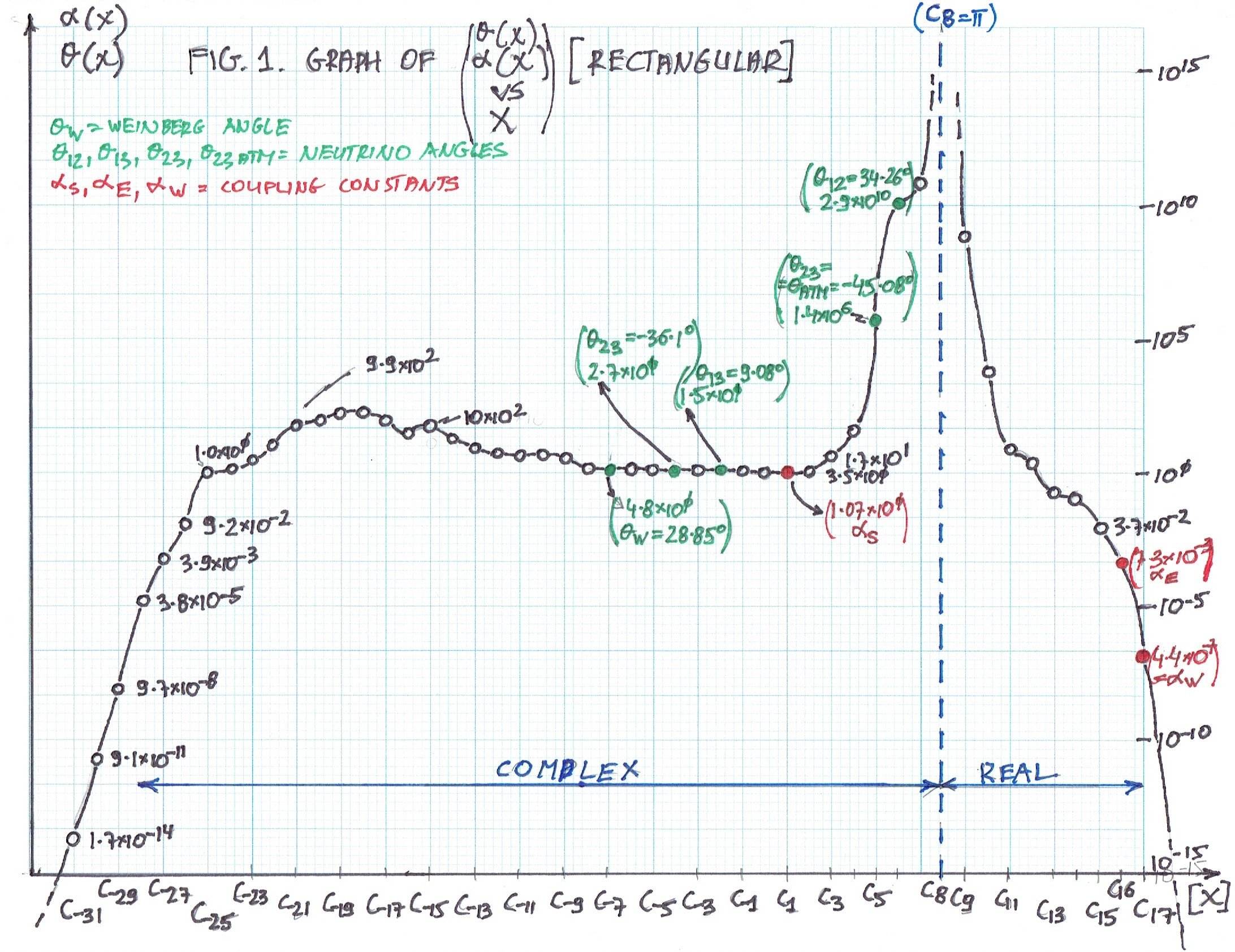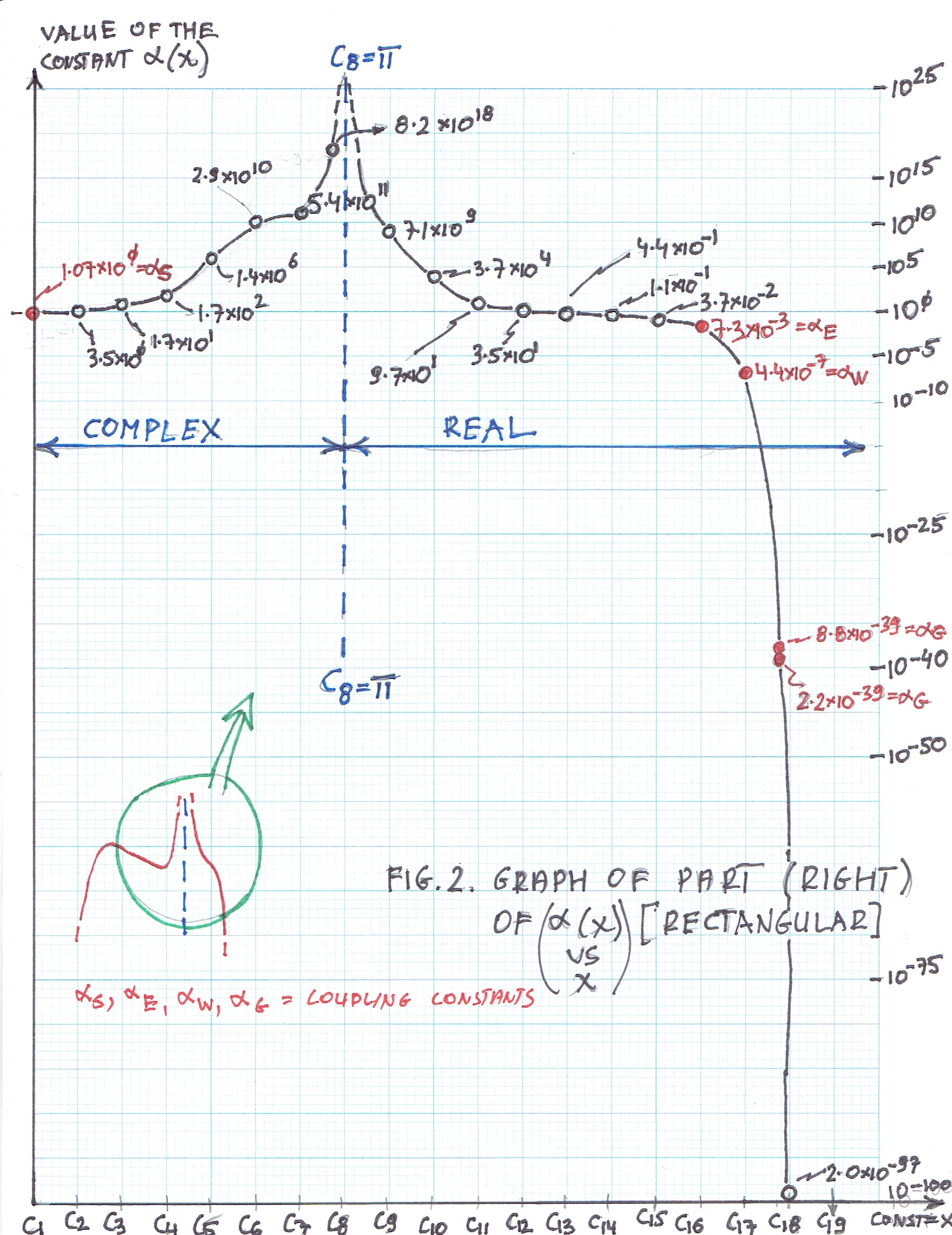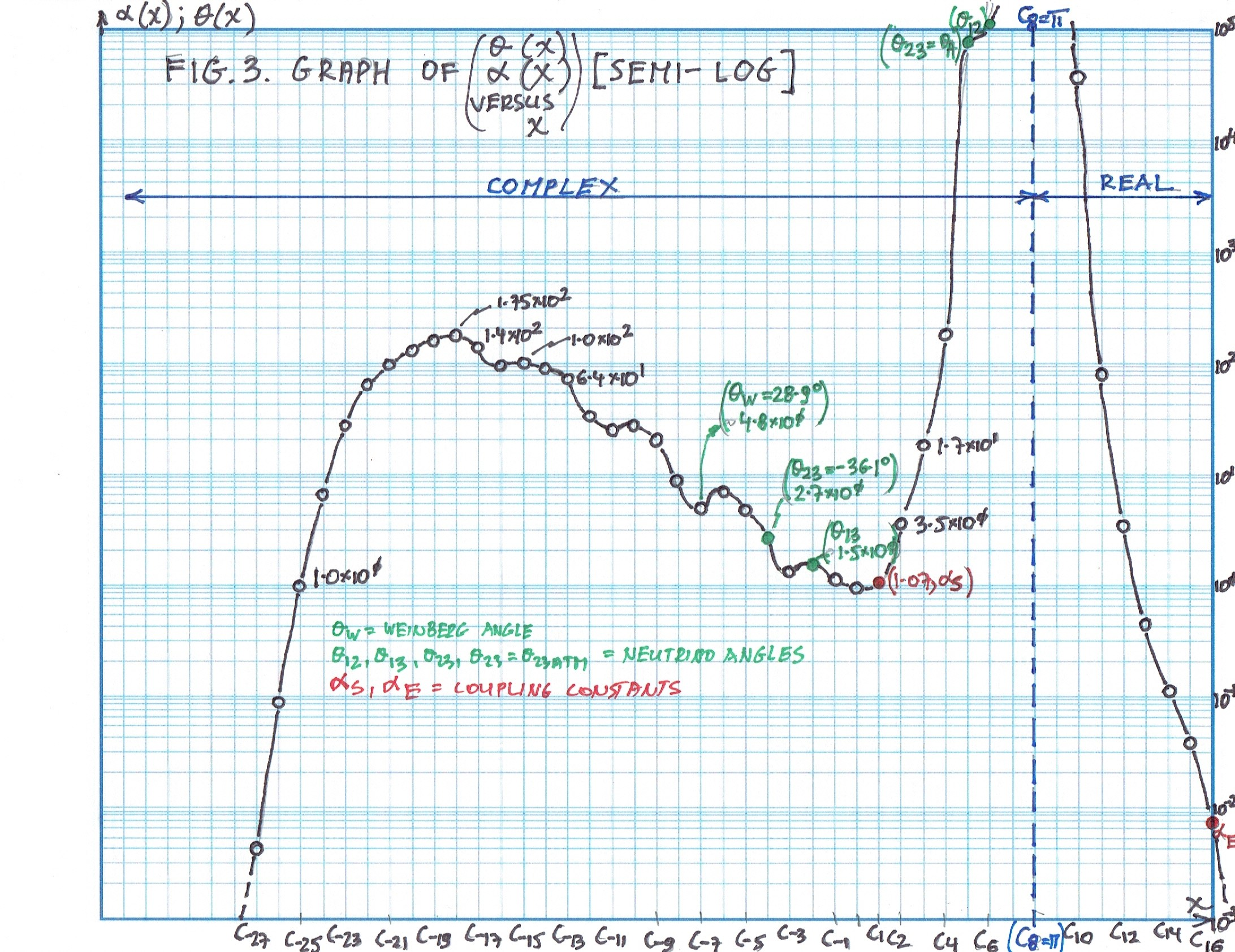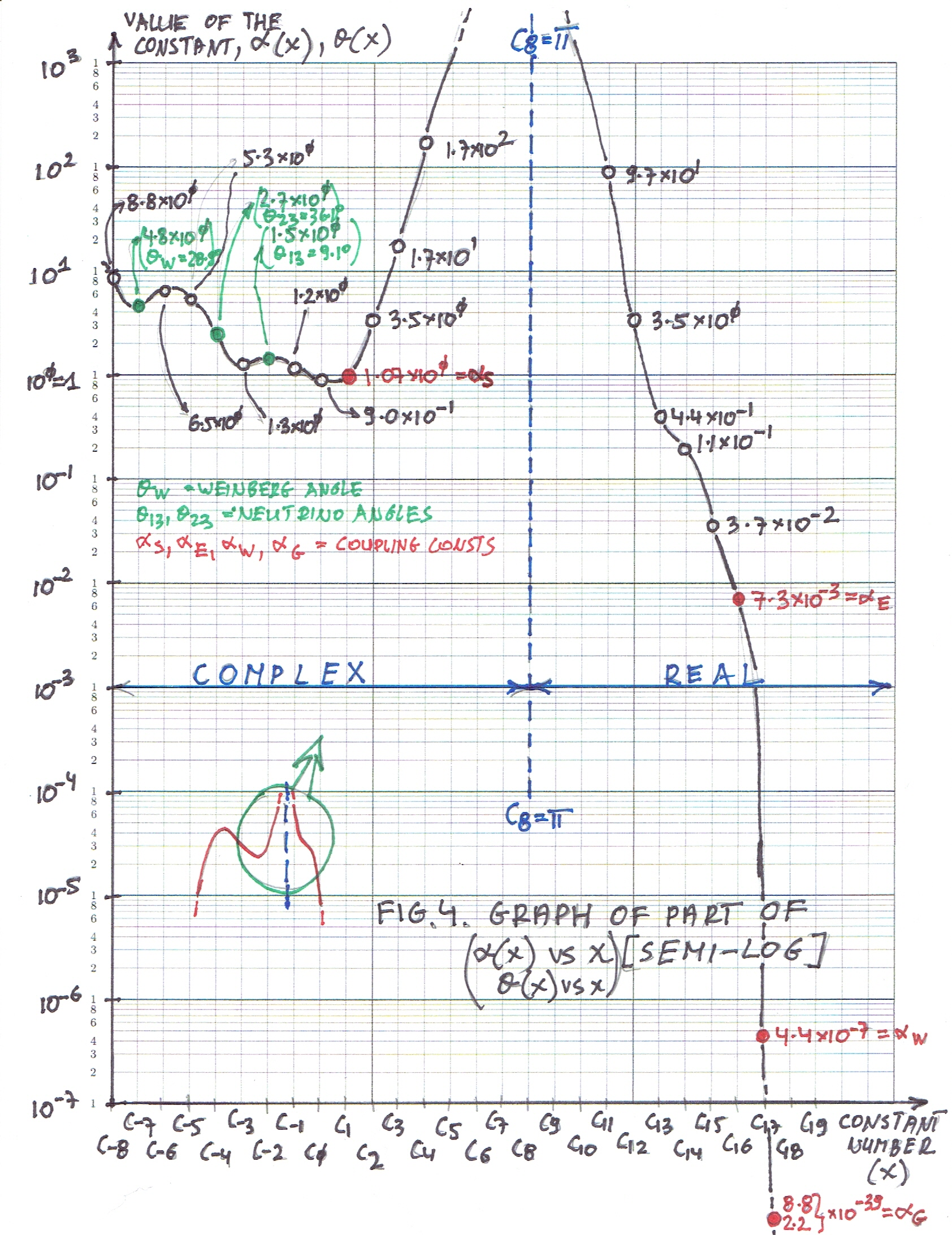## Book 7 - Here are the Graphs Only  II

6 October 2017 AD Feast of St Bruno

Andrew Yanthar-Wasilik

Here are some graphs with short descriptions.

Graph 1 - Figure 1 - Rectangular. Showing the function alpha vs constant, and theta vs constant.

Graph 2 - Figure 2 - Rectangular. Showing right part of Graph 1 - Figure 1.

Graph 3 - Figure 3 - Semi-log. Showing function alpha vs constant, and theta vs constant. Similar to Graph 1 - Figure 1.

Graph 4 Figure 4 - Semi-log. Showing right part of Graph 3 - Figure 3.

Marked in red are coupling constants, in green - neutrino mixing angles.

Andrew Yanthar-Wasilik